Next: 5.5 Contact Model Up: 5.4 Boundaries Previous: 5.4.1 Neumann Type

## 5.4.2 Dirichlet Type

For Dirichlet boundary conditions one gets
 f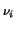=- h(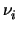) = 0 (5.18) fFC = FC +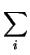fS= 0 (5.19)

Here,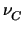is the boundary value of the quantity, which is a solution variable, whereas (5.19) is used as constitutive relation for the actual flow over the boundary FC.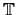B reads, again for two example grid points i1 and i2

 tx, y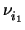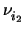FCFC 1 1

The rows forandare zero since substitute equations will directly be provided in the boundary matrixB.

Tibor Grasser
1999-05-31